# Part 3 — Titanic, extracting the features and model building

As you read on part 2 here, it was not clever to feed all the features inside the model and wait for the good results. Well, I was not clever and I did it but I think it was a necessary part of my learning curve. I realized that I need to see more effort with data processing if I want to train a better model.

There are many ways to processes the data and this is a post about how I did it.

As always I started with basic things as loading the packages, reading in the CSV-file, etc. I will skip this part in this post.

Let´s start with the missing values

`df.isnull().sum()# Count missing values in each columndf['Age'] = df['Age'].fillna((df['Age'].mean()))# replace missing values with the average values in column "age"df["Embarked"].fillna( method ='ffill', inplace = True)# replace missing values with last value in column "Embarked"df["Cabin"].fillna("Empty", inplace = True)# all missing rows are filled with word "empty" in column "cabin"`

I realized I have many columns that are not giving any information. For example, passenger ID or “Ticket”. So I decide to drop those columns. Also, I deleted “Cabin” column because most of the rows in the “Cabin” column were empty.

`df=df.drop(columns=["Ticket"])df=df.drop(columns=["Cabin"])df=df.drop(columns=["PassengerId"])df.head()`

I wanted to extract prefix of names into separate columns so that I can use it in model building.

`df1 = pd.DataFrame(df.Name.str.split(' ',1).tolist(),                                   columns = ['First','Last'])df1.head()`
`df1= pd.DataFrame(df1.Last.str.split(' ',1).tolist(),                                   columns = ['prefix','Last'])df1.head()`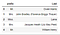Prefixes are in a separate column

Then I added prefix to df.

`df['prefix']=df1.prefixdf.head()`

The next step was to look through the prefixes.

`df['prefix'].value_counts()`

The result was not what I expected.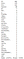There are values which should be cleaned

So all prefixes which ended with a comma I renamed to be as “-”.

`df['prefix']=np.where(df.prefix.str.endswith('.'), df.prefix, '_')df['prefix'].value_counts()`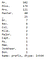The result is much better

I choose to rename as “other” which frequency was less than 25.

`prefix_count=df['prefix'].value_counts()prefix_replace_list=list(prefix_count[prefix_count<=25].index)df['prefix']=np.where(df.prefix.isin(prefix_replace_list), "other", df.prefix)df['prefix']`

Also now it was time to drop the “name” column because it is not giving us any worthy information.

`df=df.drop(columns=["Name"])`

Then the next step was to transform all the values to numbers so that the model can really use the data.

`df = pd.get_dummies(df, prefix_sep='_', drop_first=True)df.head()`

This was the file I saved for model building.

`df.to_excel("to_model_building.xlsx")`

Then I wanted to try if the model accuracy was better after extracting more features.

And the results were much better!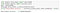Now the accuracy is 77%, earlier it was only 46%!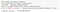73% -> 79%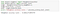58% ->62%

GaussianNB model gets much better but still, the LinearSVC model was the best.

I hope this opened your eyes to how important it is to play with the data and how much it helps to improve the model accuracy.

Thank you for the highly trained monkey (Risto Hinno) for motivating and inspiring me!